# Vienna Elections 2020: Age profile of electoral candidates

An empirical look at candidates’ age.
Austria
elections
OCR
regex
reactable
gt
Author

Roland Schmidt

Published

10 Oct 2020

# 1 Setup

Code
``````library(tidyverse)
library(here)
library(extrafont)
loadfonts(device = "win", quiet = T)
library(hrbrthemes)
hrbrthemes::update_geom_font_defaults(
family = "Roboto Condensed",
size = 3.5,
color = "grey50"
)
library(scales)
library(knitr)
library(paletteer)
library(ggtext)
library(glue)
library(pdftools)
library(svglite)
library(tictoc)
library(tidytext)
library(gt)
library(reactable)
library(reactablefmtr)
library(ggforce)
library(ggiraph)
library(htmltools)``````
Code: Define rmarkdown options
Code
``````knit_hooks\$set(wrap = function(before, options, envir) {
if (before) {
paste0("<", options\$wrap, ">")
} else {
paste0("</", options\$wrap, ">")
}
})

knitr::opts_chunk\$set(
fig.align = "left",
message = FALSE,
warning = FALSE,
dev = "svglite",
#   dev.args = list(type = "CairoPNG"),
dpi = 300,
out.width = "100%"
)
options(width = 180, dplyr.width = 150)``````
Code: Define plot theme, party colors, caption
Code
``````plot_bg_color <- "white"

caption_table <- "Source:\ndata: https://www.wien.gv.at/politik/wahlen/grbv/2020/ analysis: Roland Schmidt | @zoowalk | https://werk.statt.codes"

theme_post <- function() {
hrbrthemes::theme_ipsum_rc() +
theme(
plot.background = element_rect(fill = plot_bg_color, color=NA),
panel.background = element_rect(fill = plot_bg_color, color=NA),
#panel.border = element_rect(colour = plot_bg_color, fill=NA),
#plot.border = element_rect(colour = plot_bg_color, fill=NA),
plot.margin = ggplot2::margin(l = 0,
t = 0.25,
unit = "cm"),
plot.title = element_markdown(
color = "grey20",
face = "bold",
margin = ggplot2::margin(l = 0, unit = "cm"),
size = 11
),
plot.title.position = "plot",
plot.subtitle = element_text(
color = "grey50",
margin = ggplot2::margin(t = 0.2, b = 0.3, unit = "cm"),
size = 10
),
plot.caption = element_text(
color = "grey50",
size = 8,
hjust = c(0)
),
plot.caption.position = "panel",
axis.title.x = element_text(
angle = 0,
color = "grey50",
hjust = 1
),
axis.text.x = element_text(
size = 9,
color = "grey50"
),
axis.title.y = element_blank(),
axis.text.y = element_text(
size = 9,
color = "grey50"
),
panel.grid.minor.x = element_blank(),
panel.grid.major.x = element_blank(),
panel.grid.minor.y = element_blank(),
panel.spacing = unit(0.25, "cm"),
panel.spacing.y = unit(0.25, "cm"),
strip.text = element_text(
angle = 0,
size = 9,
vjust = 1,
face = "bold"
),
legend.title = element_text(
color = "grey30",
face = "bold",
vjust = 1,
size = 7
),
legend.text = element_text(
size = 7,
color = "grey30"
),
legend.justification = "left",
legend.box = "horizontal", # arrangement of multiple legends
legend.direction = "vertical",
legend.margin = ggplot2::margin(l = 0, t = 0, unit = "cm"),
legend.spacing.y = unit(0.07, units = "cm"),
legend.text.align = 0,
legend.box.just = "top",
legend.key.height = ggplot2::unit(0.2, "line"),
legend.key.width = ggplot2::unit(0.5, "line"),
text = element_text(size = 5)
)
}

data_date <- format(Sys.Date(), "%d %b %Y")

my_caption <- glue::glue("data: https://www.wien.gv.at/politik/wahlen/grbv/2020/\nanalysis: Roland Schmidt | @zoowalk | https://werk.statt.codes")``````

# 2 Context

Elections in Vienna are today and while glancing through the electoral lists I couldn’t help but paying attention to candidates’ birth years. Maybe that’s an age thing… This got me thinking that I haven’t seen any more systematic analysis of parties’/candidates’ age profile. So as a modest contribution to this end, here are my two cents. Again, I’ll focus mainly on the pertaining steps in R and related number crunching. Due to a lack of time and not being an expert on Vienna’s electoral system, I’ll be brief when it comes to substantive matters. But the presented results hopefully provide sufficient material to dig into.

As always, if you see any glaring error or have any constructive comment, feel free to let me now (best via twitter DM).

# 3 Data

Again, as so often, the trickiest part is to get the data ‘liberated’ from the format it is provided in. The entire list of candidates is published in this pdf. Note that there are three lists: One for the city council (‘Gemeinderat’; composed on the basis of the results in 18 multi-member electoral districts), one for the 23 district councils (‘Bezirksrat’; one in each district), and the ‘city election proposal’ (‘Stadtwahlvorschlag’; admittedly a somewhat clumsy translation). The latter doesn’t constitute a body in itself, but serves to allocate mandates which remained unassigned after counting the votes for the city council (‘zweites Ermittlungsverfahren’/similar to the d’Hondt procedure).

When it comes to extracting the data from the linked pdf, a difficulty may arise due to the two-column format of the document. Hence, simple row-wise extraction doesn’t help much since it would put candidates together which could be from different parties. Similarly, simply isolating the two columns and extracting candidates would also not do the trick since breaks betwee parties, districts etc run over two, and not one column. To illustrate this, I resorted to cutting edge technology and drew the two arrows below:

Luckily, the `tabulizer` package is not only very powerful when it comes to extracting text/data from a pdf, it is also sophisticated enough to take into consideration the text flow highlighted above. I am not familiar with the underlying heuristic, but I assume it is contingent on consistently formatted section headings. Hence, empowered with this tool, retrieving the text becomes rather effortless. The subsequent steps are a battery of regular expressions to extract the specific data we are interested in. To see the code, unfold the snippets below.

Code: Extract text from pdf
Code
``````df_raw <- tabulizer::extract_text(file=here::here("_blog_data",
"vienna_elections_2020",
"amtsblatt2020.pdf"),
pages=c(1:115),
encoding="UTF-8") %>%  #capital letters of UTF!
enframe(name=NULL,
value="text_raw")``````
Code: Extract data of interest (regex)
Code
``````df_clean <- df_raw %>%
ungroup() %>%
mutate(text_split=str_split(text_raw, regex("\r\n\\s*(?=\\d+\\.)"))) %>%
unnest_longer(text_split) %>%
mutate(text_split=text_split %>% str_squish() %>% str_trim()) %>%
mutate(text_split=str_split(text_split, ".(?=Zustellungsbevollmächtigte(r)? Vertreter(in)?)")) %>%
unnest_longer(text_split)

# get Listenplatz ------------------------------------------------------------

df_clean <- df_clean %>%
mutate(listenplatz=str_extract(text_split, regex("^\\d+\\.?\\s+(?!Bezirk)")) %>%
str_extract(., "\\d*") %>% as.numeric())

# get elections -----------------------------------------------------------

df_clean <- df_clean %>%
mutate(election=text_raw %>% str_extract(., regex("(?<=[A-Z]\\.)\\s*[A-z]+wahl(en)?", dotall = T)) %>%
str_trim(., side=c("both"))) %>%
tidyr::fill(election, .direction="down")

# electoral district --------------------------------------------------------------
df_clean <- df_clean %>%
mutate(wahlkreis=case_when(election=="Bezirksvertretungswahlen" ~ str_extract(text_split, "\\d{1,2}\\. Bezirk"),
election=="Gemeinderatswahl"~ str_extract(text_split, regex("Wahlkreis.*?(?=[:upper:]{2,}?)",
dotall = T,
multiline = T)),
TRUE ~ as.character("missing"))) %>%
mutate(wahlkreis=str_trim(wahlkreis, side=c("both"))) %>%
tidyr::fill(wahlkreis, .direction="down") %>%
mutate(wahlkreis=str_remove(wahlkreis, "Wahlkreis ") %>%
str_remove(., regex("\\(.*\\)")) %>%
str_trim(., side=c("both")))

# other -------------------------------------------------------------------

df_clean <- df_clean %>%
mutate(page=text_raw %>% str_extract(., regex("Seite \\d+")) %>% str_extract(., "\\d+") %>%
as.numeric()) %>%
mutate(name=str_extract(text_split, regex("(?<=\\d\\.\\s?).*?(?=,\\s?\\d{4},)")) %>%
str_trim(., side=c("both"))) %>%
mutate(first_name=text_split %>% str_extract(., regex("[:alpha:]*(?=,\\s?\\d+)"))) %>%
mutate(year_birth=text_split %>% str_extract(., regex("\\d{4}")) %>%
as.numeric()) %>%
mutate(year_interval=cut(year_birth, seq(1930, 2005, 5))) %>%
mutate(plz=text_split %>% str_extract(., regex("\\d{4}\\s(?=Wien)")) %>%
str_trim(., side=c("both")))

# get party ---------------------------------------------------------------

df_clean <- df_clean %>%
mutate(party=text_split %>% str_extract(., regex("(?<=^Zustellung)[:alpha:]*\$"))) %>%
TRUE ~ NA_character_)) %>%
tidyr::fill(party, .direction = "down") %>%
mutate(party=party %>%
as_factor() %>%
fct_relevel(., sort) %>%
fct_relevel(., "SPÖ", "FPÖ", "GRÜNE", "ÖVP", "NEOS"))

# wrap up -----------------------------------------------------------------

df_clean <- df_clean %>%
mutate(wahlkreis_plz=str_extract(wahlkreis, regex("\\d+")) %>%
as.numeric()+100) %>%
mutate(wahlkreis_plz=wahlkreis_plz %>% as.character() %>% paste0(., "0")) %>%
mutate(wahlkreis_plz=case_when(str_detect(wahlkreis, "Zentrum") ~ "1010, 1040, 1050, 1060",
str_detect(wahlkreis, "Innen") ~ "1070, 1080, 1090",
str_detect(wahlkreis, "Landstraße") ~ "1030",
str_detect(wahlkreis, "Favoriten") ~ "1100",
str_detect(wahlkreis, "Simmering") ~ "1110",
str_detect(wahlkreis, "Meidling") ~ "1120",
str_detect(wahlkreis, "Hietzing") ~ "1130",
str_detect(wahlkreis, "Penzing") ~ "1140",
str_detect(wahlkreis, "Rudolf") ~ "1150",
str_detect(wahlkreis, "Ottakring") ~ "1160",
str_detect(wahlkreis, "Hernals") ~ "1170",
str_detect(wahlkreis, "Währing") ~ "1180",
str_detect(wahlkreis, "Döbling") ~ "1190",
str_detect(wahlkreis, "Brigittenau") ~ "1200",
str_detect(wahlkreis, "Floridsdorf") ~ "1210",
str_detect(wahlkreis, "Liesing") ~ "1230",
TRUE ~ as.character(wahlkreis_plz))) %>%
mutate(residence=case_when(
str_detect(wahlkreis_plz, plz) ~ "inside",
!str_detect(wahlkreis_plz, plz) ~ "outside",
TRUE ~ as.character("missing"))) #%>%

df_clean <- df_clean %>%
select(-text_raw) %>%
filter(!is.na(listenplatz)) ``````

After these few steps we have a searchable/sortable table of all candidates (or better candidatures since one person can be candidate on multiple districts/lists). There have been 8,983 candidatures by 5,038 individuals.

The table essentially provides all necessary data for the subsequent analysis. While the pdf does not include the exact birth date of each candidate, it provides us with their birth years which we can take as a proxy for age. Note that I also extracted candidates’ residence zip code to see how often place of residence and candidature actually overlap (see below).

## 3.1 Result

Code: Table with all candidates
Code
``````tb_all <- reactable(df_clean %>%
select(election, wahlkreis, party, name, listenplatz, year_birth, plz),
columns=list(election=colDef(name="Wahl", width=130),
wahlkreis=colDef(name="Wahlbezirk", width=100),
party=colDef(name="Partei", width=50),
name=colDef(name="KandidatIn"),
listenplatz=colDef(name="Listenplatz",
width=70,
align="center"),
year_birth=colDef(name="Geburtsjahr", width=90),
plz=colDef(name="PLZ Wohnort", width=90)),
bordered=F,
compact = TRUE,
highlight = TRUE,
style = list(fontSize = "10px"),
filterable = TRUE,
defaultPageSize = 23,
theme = reactablefmtr::nytimes()) %>%
add_title(title= "WIEN-WAHL 2020: Liste aller KandidatInnen") %>%

# WIEN-WAHL 2020: Liste aller KandidatInnen

Source: data: https://www.wien.gv.at/politik/wahlen/grbv/2020/ analysis: Roland Schmidt | @zoowalk | https://werk.statt.codes

# 4 Analysis

## 4.1 Oldest and youngest candidates

Let’s now look at the overall youngest and oldest candidates. We could retrieve this information already from the main table provided above (sort column birth year). Here, however, let’s nest candidates’ different candidatures for the sake of clarity.

### 4.1.1 Youngest candidates

Code: Youngest candidates
Code
``````df_main <- df_clean %>%
distinct(name, year_birth, party) %>%
slice_max(.,order_by=year_birth, n=10) %>%
arrange(name, desc(year_birth)) %>%
mutate(index=dplyr::min_rank(year_birth)) %>%
mutate(index_name=paste(index, ". ",name), .before=1) %>%
select(-index)

tb_young <- reactable(df_main,
columns=list(index_name=colDef(name="KandidatIn",
width=130),
name=colDef(show=F),
year_birth=colDef(name="Geburtsjahr",
width=70),
party=colDef(name="Partei",
width=50)),
pagination = FALSE,
onClick = "expand",
bordered=F,
compact = TRUE,
highlight = TRUE,
rowStyle = list(cursor = "pointer"),
style = list(fontSize = "10px"),
theme = reactableTheme(
borderWidth = 1,
borderColor = "#7f7f7f",
backgroundColor = plot_bg_color,
filterInputStyle = list(
color="green",
backgroundColor = plot_bg_color)),

details=function(index){
df_nested <- df_clean %>%
slice_max(.,order_by=year_birth, n=10) %>%
select(name, election, wahlkreis, listenplatz) %>%
filter(name==df_main\$name[index]) %>%
select(-name)

tbl_nested <- reactable(df_nested,
columns = list(
election=colDef(name="Wahl"),
wahlkreis=colDef(name="Wahlbezirk"),
listenplatz=colDef(name="Listenplatz")
),
outlined = TRUE,
highlight = TRUE,
fullWidth = TRUE,
theme = reactableTheme(
backgroundColor = "#ab8cab"))

htmltools::div(style = list(margin = "12px 45px"), tbl_nested)}

) %>%
add_source(source="Geburtsjahr lt. Wahlvorschlag als Basis. Top 10.")``````

# WIEN-WAHL 2020: Jüngesten KandidatInnen

Geburtsjahr lt. Wahlvorschlag als Basis. Top 10.

As becomes clear from the table, there are overall 16 candidates who were all born in 2002.

### 4.1.2 Oldest candidates

Code: oldest candidates
Code
``````df_main <- df_clean %>%
distinct(name, year_birth, party) %>%
slice_min(., order_by=year_birth, n=10) %>%
arrange(year_birth, name) %>%
mutate(index=dplyr::min_rank(year_birth)) %>%
mutate(index_name=paste(index, ". ", name), .before=1) %>%
select(-index)

tb_old <- reactable(df_main,
columns=list(index_name=colDef(name="KandidatIn",
width=130),
name=colDef(show=F),
year_birth=colDef(name="Geburtsjahr",
width=70),
party=colDef(name="Partei",
width=50)),
pagination = FALSE,
onClick = "expand",
bordered=F,
compact = TRUE,
highlight = TRUE,
rowStyle = list(cursor = "pointer"),
style = list(fontSize = "10px"),
theme = reactableTheme(
borderWidth = 1,
borderColor = "#7f7f7f",
backgroundColor = plot_bg_color,
filterInputStyle = list(
color="green",
backgroundColor = plot_bg_color)),

details=function(index){
df_nested <- df_clean %>%
slice_min(.,order_by=year_birth, n=10) %>%
select(name, election, wahlkreis, listenplatz) %>%
filter(name==df_main\$name[index]) %>%
select(-name)

tbl_nested <- reactable(df_nested,
columns = list(
election=colDef(name="Wahl"),
wahlkreis=colDef(name="Wahlbezirk"),
listenplatz=colDef(name="Listenplatz")
),
outlined = TRUE,
highlight = TRUE,
fullWidth = T,
theme = reactableTheme(
backgroundColor = "#ab8cab"))

htmltools::div(style = list(margin = "12px 45px"), tbl_nested)}

) %>%
add_source(source="Geburtsjahr lt. Wahlvorschlag als Basis. Top 10.")``````

# WIEN-WAHL 2020: Ältesten KandidatInnen

Geburtsjahr lt. Wahlvorschlag als Basis. Top 10.

The oldest candidate is Waschiczek Wolfgang, who was born in 1928. Not bad.

## 4.2 Avgerage birth year per election and party

Let’s now look at the average year of birth of parties’ candidates on each of the different electoral levels. The table below provides the median, mean and standard deviation for each party. The thin white line in the density plots on the right indicates the median.

Code: Median age of lists
Code
``````# summarize data (median, mean, sd)
df_list_age <- df_clean %>%
group_by(election, party) %>%
summarize(year_median=median(year_birth, na.rm = T),
year_mean=mean(year_birth, na.rm=T),
year_sd=sd(year_birth, na.rm=T)) %>%
group_by(election) %>%
arrange(desc(year_median), .by_group=T) #order as gt table

#create graphs for table

## define function creating plot
fn_plot <- function(data){
data %>%
ggplot()+
ggridges::geom_density_ridges(aes(x=year_birth,
y=0),
fill="firebrick",
color=plot_bg_color,
quantile_lines=T,
quantiles=2,
panel_scaling = F,
linewidth=12)+
scale_x_continuous(limits=c(min(df_clean\$year_birth),
max(df_clean\$year_birth)),
expand=expansion(mult=0))+
scale_y_discrete(expand=expansion(mult=0))+
theme(
plot.background = element_rect(fill = plot_bg_color, color=NA),
panel.background = element_rect(fill = plot_bg_color, color=NA),
plot.margin = ggplot2::margin(0, unit="cm"),
axis.text = element_blank(),
axis.title = element_blank()
)

}

## apply function, dataframe with plots
box_plot <- df_clean %>%
select(election, party, year_birth) %>%
group_by(election, party) %>%
mutate(year_median=median(year_birth, na.rm = T)) %>%
#ungroup() %>%
nest(year_birth_nest=c(year_birth)) %>%
mutate(plot=map(year_birth_nest, fn_plot)) %>%
group_by(election) %>%
arrange(desc(year_median), .by_group=T) #order as gt table

#create gt table & insert df with plots;
tb_list_age <- df_list_age %>%
mutate(year_mean=round(year_mean, digits = 2)) %>%
select(election, party, contains("year")) %>%
group_by(election) %>%
arrange(desc(year_median), .by_group=T) %>% #order as plots
mutate(index=min_rank(-year_median), .before=1) %>%
mutate(index_party=paste0(index,". ", party)) %>%
ungroup() %>%
select(-index, -party) %>%
mutate(boxplot=NA) %>%
gt(groupname_col = "election",  rowname_col  = "index_party") %>%
tab_header(title=md("**WIEN-WAHLEN 2020:<br>Durchschnittliches Geburtsjahr der KandidatInnen**")) %>%
gt::cols_label(index_party="Partei",
year_median="Median",
year_mean="arith. Mittel",
year_sd="Std. Abw.",
boxplot="Verteilung") %>%
gt::fmt_number(columns=c(year_sd),
decimals=2,
suffixing=F) %>%
gt::text_transform(
locations=cells_body(c(boxplot)),
fn=function(x){
map(box_plot\$plot, ggplot_image, height=px(11), aspect_ratio=4)}
) %>%
cols_width(
c(boxplot) ~ px(200),
c(index_party) ~ px(100)
) %>%
table.background.color = plot_bg_color,
table.width=pct(100),
table.font.size = "11px",
row_group.font.weight = "bold",
 Median arith. Mittel Std. Abw. WIEN-WAHLEN 2020:Durchschnittliches Geburtsjahr der KandidatInnen 1991.5 1992.12 2.85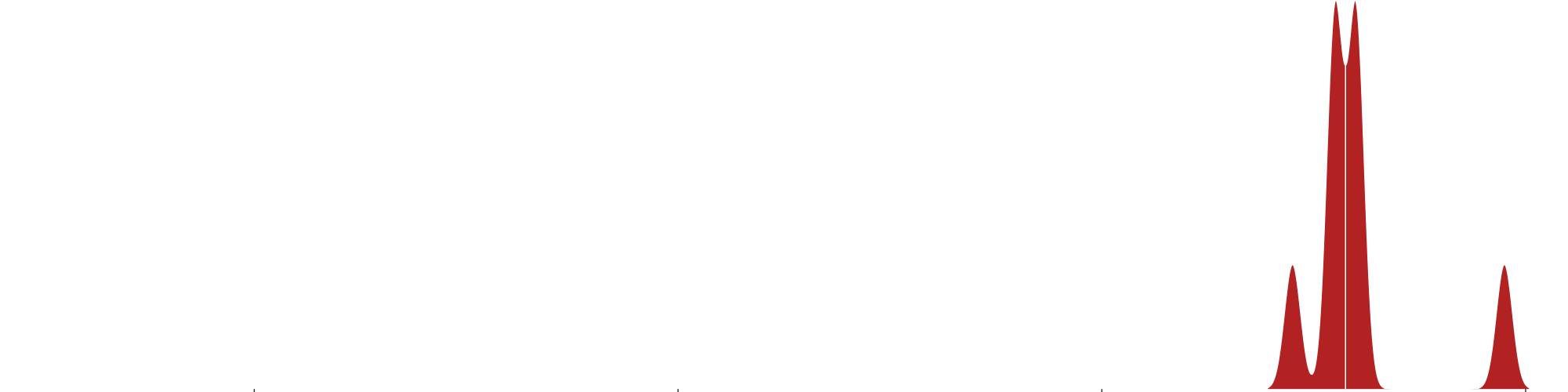1988.0 1985.63 10.57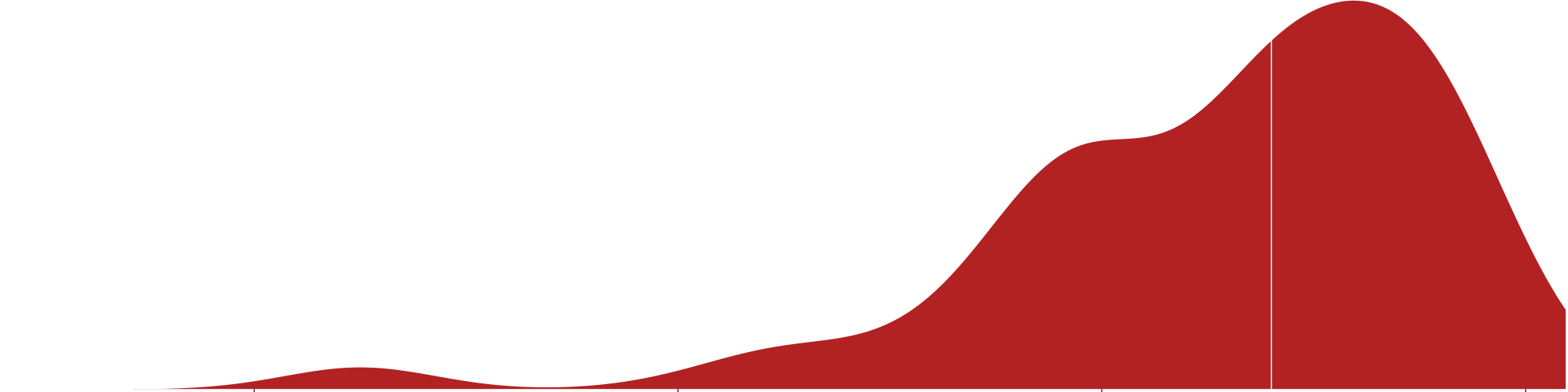1986.0 1984.50 5.83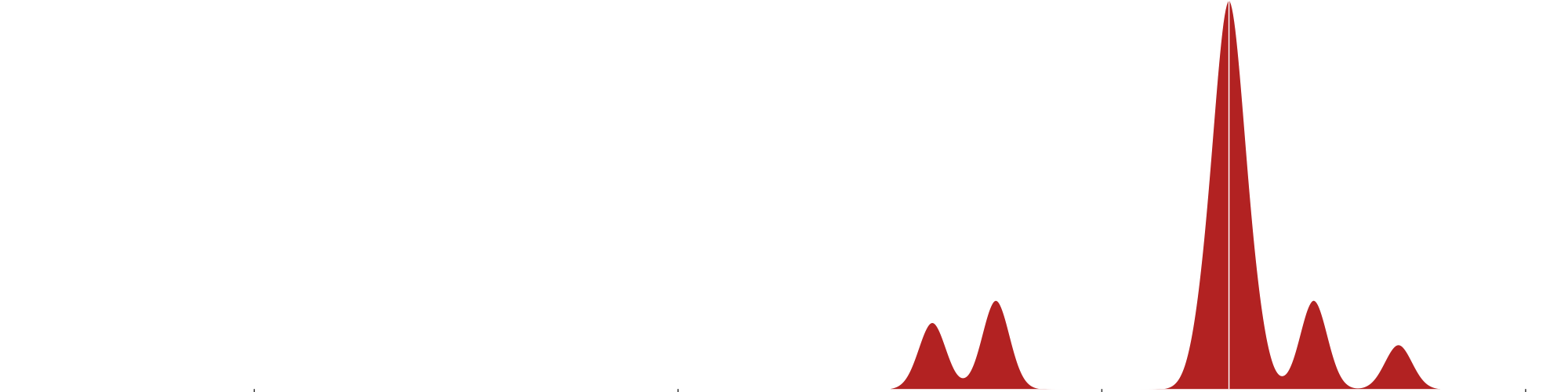1983.0 1978.02 16.36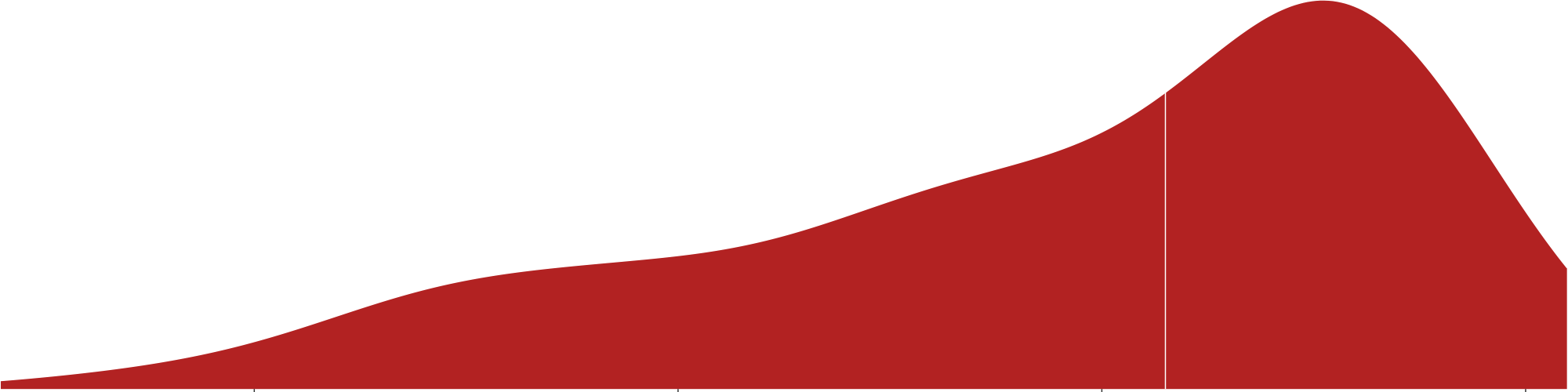1981.0 1975.00 26.751977.0 1977.59 13.201976.0 1975.66 12.421975.0 1975.26 16.061973.0 1973.43 12.83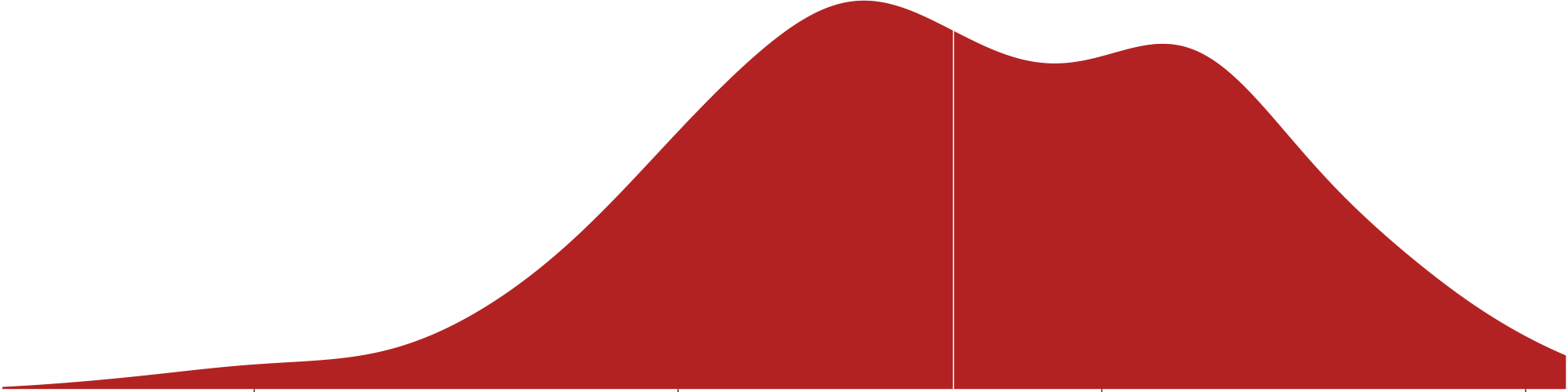1970.0 1971.81 14.96